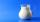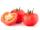# Fraction calculator

The calculator performs basic and advanced operations with fractions, expressions with fractions combined with integers, decimals, and mixed numbers. Also shows detailed step-by-step information about fraction calculation procedure. Solve problems with two, three or more fractions and numbers in one expression.

## Result:

### How do you solve fractions step by step?

1. Multiple: 38 * 100 = 3 · 1008 · 1 = 3008 = 75 · 42 · 4 = 752
Multiply both numerators and denominators. Result fraction keep to lowest possible denominator GCD(300, 8) = 4. In the next intermediate step cancelling by a common factor of 4 gives 752.
In words - three eighths multiplied by one hundred = seventy-five halfs.

#### Rules for expressions with fractions:

Fractions - use the slash “/” between the numerator and denominator, i.e. for five-hundredths enter 5/100. If you are using mixed numbers be sure to leave a single space between the whole number and fraction part.
The slash separates the numerator (number above a fraction line) and denominator (number below).

Mixed numerals (mixed fractions or mixed numbers) write as non-zero integer separated by one space and fraction i.e., 1 2/3 (having the same sign). An example of a negative mixed fraction: -5 1/2.
Because slash is both signs for fraction line and division, we recommended use colon (:) as operator of division fractions i.e., 1/2 : 3.

Decimals (decimal numbers) enter with a decimal point . and they are automatically converted to fractions - i.e. 1.45.

Colon : and slash / is the symbol of division. Can be used to divide mixed numbers 1 2/3 : 4 3/8 or can be used for write complex fractions i.e. 1/2 : 1/3.
An asterisk * or × is the symbol for multiplication.
Plus + is addition, minus sign - is subtraction and ()[] is mathematical parentheses.
The exponentiation/power symbol is ^ - for example: (7/8-4/5)^2 = (7/8-4/5)2

#### Examples:

subtracting fractions: 2/3 - 1/2
multiplying fractions: 7/8 * 3/9
dividing Fractions: 1/2 : 3/4
exponentiation of fraction: 3/5^3
fractional exponents: 16 ^ 1/2
adding fractions and mixed numbers: 8/5 + 6 2/7
dividing integer and fraction: 5 ÷ 1/2
complex fractions: 5/8 : 2 2/3
decimal to fraction: 0.625
Fraction to Decimal: 1/4
Fraction to Percent: 1/8 %
comparing fractions: 1/4 2/3
multiplying a fraction by a whole number: 6 * 3/4
square root of a fraction: sqrt(1/16)
reducing or simplifying the fraction (simplification) - dividing the numerator and denominator of a fraction by the same non-zero number - equivalent fraction: 4/22
expression with brackets: 1/3 * (1/2 - 3 3/8)
compound fraction: 3/4 of 5/7
fractions multiple: 2/3 of 3/5
divide to find the quotient: 3/5 ÷ 2/3

Calculator follows well-known rules for order of operations. Most common mnemonics for remembering this order of operations are:
PEMDAS - Parentheses, Exponents, Multiplication, Division, Addition, Subtraction.
BEDMAS - Brackets, Exponents, Division, Multiplication, Addition, Subtraction
BODMAS - Brackets, Of or Order, Division, Multiplication, Addition, Subtraction.
GEMDAS - Grouping Symbols - brackets (){}, Exponents, Multiplication, Division, Addition, Subtraction.
Be careful, always do multiplication and division before addition and subtraction. Some operators (+ and -) and (* and /) has the same priority and then must evaluate from left to right.

## Fractions in word problems:

• PearsThere were pears in the basket, I took two-fifths of them, and left six in the basket. How many pears did I take?
• Simple equation 5Solve equation with fractions: X × 3/8 = 1/2
• Unknown numberI think the number - its sixth is 3 smaller than its third.
• WithdrawalIf I withdrew 2/5 of my total savings and spent 7/10 of that amount. What fraction do I have in left in my savings?
• Ratio 11Simplify this ratio 10 : 1/4
• Watching TVOne evening 2/3 students watch TV. Of those students, 3/8 watched a reality show. Of the students that watched the show, 1/4 of them recorded it. What fraction of the students watched and recorded reality tv.
• Cupcakes 2Susi has 25 cupcakes. She gives 4/5. How much does she have left?
• CakesOn the bowl were a few cakes. Jane ate one-third of them, Dana ate a quarter of those cakes that remained. a) What part (of the original number of cakes) Dana ate? b) At least how many cakes could be (initially) on thebowl?
• MilkAt the kindergarten, every child got 1/5 liter of milk in the morning and another 1/8 liter of milk in the afternoon. How many liters were consumed per day for 20 children?
• Passenger boatTwo-fifths of the passengers in the passenger boat were boys. 1/3 of them were girls and the rest were adult. If there were 60 passengers in the boat, how many more boys than adult were there?
• Farmers 2On Wednesday the farmers at the Grant Farm picked 2 barrels of tomatoes. Thursday, the farmers picked 1/2 as many tomatoes as on Wednesday. How many barrels of tomatoes did the farmers pick on Thursday?
• Write 3Write a real world problem involving the multiplication of a fraction and a whole number with a product that is between 8 and 10 then solve the problem
• 3 children3 children had to divide 4 pounds is candy. How much candy did each child get?